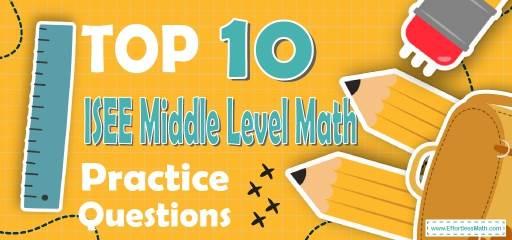# Top 10 ISEE Middle-Level Math Practice QuestionsPreparing students for the ISEE Middle-Level Math test? Looking for the most common ISEE Middle-Level Math questions and practice tests? The best way to prepare students for their ISEE Middle-Level Math test is to work through as many ISEE Middle-Level Math practice questions as possible. Here are the top 10 ISEE Middle-Level Math practice questions to help your students review the most important ISEE Middle-Level Math concepts. These ISEE Middle-Level Math practice questions are designed to cover mathematics concepts and topics that are found on the actual test. The questions have been fully updated to reflect the latest 2022 ISEE Middle-Level guidelines. Answers and full explanations are provided at the end of the post.

Help your students start their ISEE Middle-Level Math test prep journey right now with these sample ISEE Middle-Level Math questions.

## ISEE Middle Level Math Practice Questions

1- Which of the following is a correct statement?

A. $$\frac{3}{4}>0.8$$

B. $$10\%=\frac{2}{5}$$

C. $$3<\frac{5}{2}$$

D. $$\frac{5}{6}>0.8$$

2- What is the value of $$x$$ in the following figure?

A. 150

B. 145

C. 125

D. 105

3- What is the area of a square whose diagonal is 8?

A. 16

B. 32

C. 36

D. 64

4- Which of the following shows the numbers in descending order?

A. $$\frac{1}{6},\frac{2}{5},\frac{1}{3},\frac{3}{4}$$

B. $$\frac{1}{6},\frac{2}{5},\frac{3}{4},\frac{1}{3}$$

C. $$\frac{1}{6},\frac{1}{3},\frac{2}{5},\frac{3}{4}$$

D. $$\frac{1}{6},\frac{3}{4},\frac{1}{3},\frac{2}{5}$$

5- $$452,357,841×0.0001$$?

A. 452,357.841

B. 45,235.7841

C. 4,523.57841

D. 452.357841

6- A $40 shirt now selling for$28 is discounted by what percent?

A. $$20 \%$$

B. $$30 \%$$

C. $$40 \%$$

D. $$60 \%$$

7- If $$f=2x-3y$$ and $$g=x+4y$$, what is $$2f+g$$?

A. $$3x−y$$

B. $$3x−2y$$

C. $$5x−2y$$

D. $$5x−y$$

8- The area of a circle is $$64π$$. What is the diameter of the circle?

A. 4

B. 8

C. 12

D. 16

9- If there were 300 students at a school and nearly $$32\%$$ prefer to learn Germany, approximately how many students want to learn Germany?

A. 300

B. 204

C. 96

D. 6

10- What is the mean in the following set of numbers?
$$9,12,29,36,45,63,99,123$$

A. 46.2

B. 40.5

C. 59.4

D. 52

## Best ISEE Middle Level Math Prep Resource for 2022

1- D
$$\frac{5}{6}=0.83→0.8<\frac{5}{6}$$

2- B
$$x=20+125=145$$

3- B
The diagonal of the square is 8. Let $$x$$ be the side.
Use Pythagorean Theorem: $$a^2 + b^2 = c^2$$
$$x^2 + x^2 = 82 ⇒ 2x^2 = 82 ⇒ 2x^2 = 64 ⇒x^2 = 32 ⇒x= \sqrt {32}$$
The area of the square is:
$$\sqrt {32} × \sqrt{32} = 32$$

4- C
$$\frac{1}{6}≅0.16, \frac{1}{3}≅0.33, \frac{2}{5}=0.4, \frac{3}{4}=0.75$$

5- B
$$52357841×\frac{1}{10000}=45235.7841$$

6- B
Use the formula for Percent of Change
$$\frac{New \ Value \ – \ Old \ Value}{Old \ Value}× 100 \%$$
$$\frac{28-40}{40}× 100 \% = –30 \%$$
(negative sign here means that the new price is less than old price)

7- C
$$2f=2×(2x-3y)=4x-6y$$
$$2f+g=4x-6y+x+4y=5x-2y$$

8- D
The formula for the area of the circle is: $$A=πr^2$$
The area is $$64π$$. Therefore:
$$A=πr^2 ⇒ 64π = πr^2$$
Divide both sides by $$π$$:
$$64 = r^2⇒ r=8$$
Diameter of a circle is $$2 x$$ radius. Then:
Diameter $$= 2 × 8 = 16$$

9- C
Number of students prefer to learn Germany $$= \frac{32}{100}×300=96$$

10- D
Mean $$=\frac{9+12+29+36+45+63+99+123}{8}=\frac{416}{8}=52$$

Looking for the best resource to help you succeed on the ISEE Middle-Level Math test?

## The Best Books to Ace the ISEE Middle Level Math Test

### What people say about "Top 10 ISEE Middle-Level Math Practice Questions - Effortless Math: We Help Students Learn to LOVE Mathematics"?

No one replied yet.

X
23% OFF

Limited time only!

Save Over 23%

SAVE $5 It was$21.99 now it is \$16.99